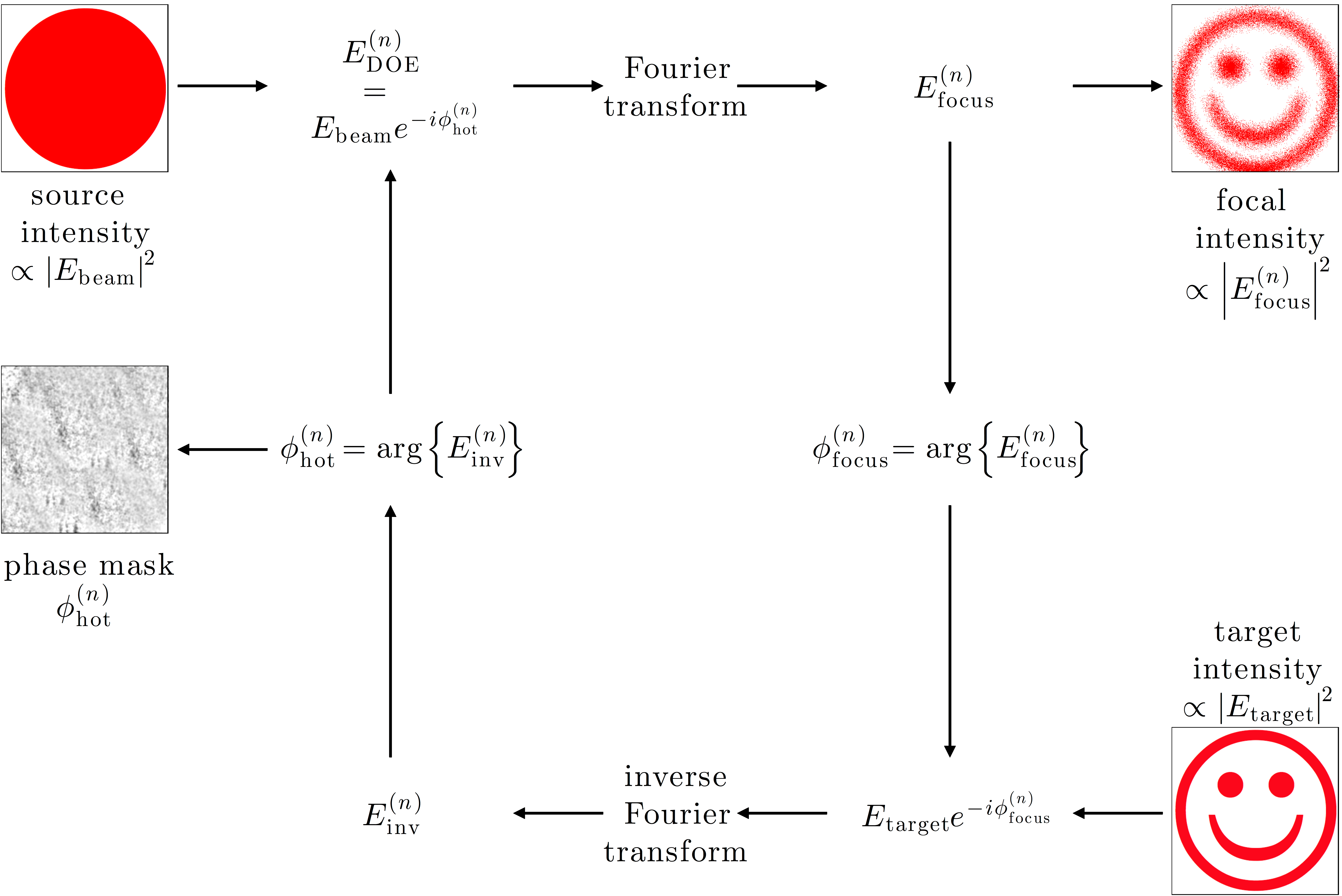# Figure 11.4 — The Gerchberg-Saxton algorithmFigure 11.4 — The Gerchberg-Saxton algorithm. Given the incoming beam electric field Ebeam, the Gerchberg–Saxton algorithm permits one to obtain a phase distribution for the hologram at the DOE plane φhot that permits one to approximate the target intensity distribution at the focal plane. At the nth iteration of the algorithm, the amplitude of the field at the DOE, EDOE(n), is given by Ebeam and its phase by φhot(n). The focal field Efocus(n) is given by the Fourier transform of EDOE(n). Next, the amplitude of the focal field is substituted with the target intensity amplitude and the resulting field is inverse Fourier transformed, obtaining Einv(n). Finally, the hologram phase is updated to the phase of Einv(n). If the focal intensity and the target intensity differ more than an acceptable error, the cycle is then repeated (until a certain maximum number of iterations is reached).